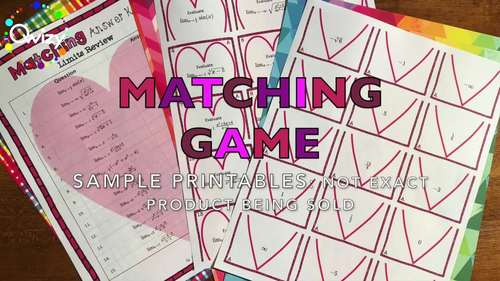# Valentine's Day Algebra BUNDLE: Matching GamesSubject
Resource Type
Product Rating
File Type

Zip

(68 MB)
7 Products in this Bundle
7 products
Bundle Description

Valentine's Day Algebra BUNDLE: Matching Games has all you need to run multiple exciting review sessions over different Algebra topics !

GET ALL OF OUR Valentine's Day MATH ALGEBRA MATCHING GAMES!!!

All of the following Algebra Matching Games are included:

1) Valentine's Day: Order of Operations Matching Game is the perfect activity for your students to sharpen their understanding of Order of Operations! Students will be asked to evaluate expressions containing addition, subtraction, multiplication, division, and powers of integers.

2) Valentine's Day: Algebra One & Two-Step Equations Matching Game is the perfect activity for your students to sharpen their understanding of solving linear equations! Students are asked to solve linear equations that contain integer and fractional constants and variables. Each equation will only need one or two steps to be solved.

3) Valentine's Day: Algebra Multi-Step Equations Matching Game is the perfect activity for your students to sharpen their understanding of solving linear equations! Students are asked to solve linear equations that contain constants and variables in the form of integers on one and both sides of the equation. Each equation will require multiple steps to be solved.

4) Valentine's Day: Systems of Equations Matching Game is the perfect activity for your students to sharpen their understanding of solving systems. The systems of linear equations include 2 variables, x & y. All solutions are integers expressed as points (x, y). Equations are written in standard form, slope intercept form, and also completely mixed up.

5) Valentine's Day: Algebra Simplifying Exponents Matching Game is the perfect activity for your students to sharpen their understanding of Exponent Rules! Expressions include monomials of one or two variables with positive or negative exponents being multiplied or divided for the students to simplify.

6) Valentine's Day: Algebra Factoring #1 Matching Game is the perfect activity for your students to sharpen their understanding of Factoring ! Quadratic Expressions reduce to linear factors. Factoring techniques required are Greatest Common Factor, Difference of Two Squares, and Trinomials with and without a coefficient in front.

7) Valentine's Day: Algebra Simplifying Polynomials #1 Matching Game is the perfect activity for your students to sharpen their understanding of Addition, Subtraction, and Multiplication of Polynomials ! Functions include monomials, binomials, and trinomials for the students to Add, Subtract, and Multiply with a maximum degree of 2.

Each of the Products listed above contains 5 Different Valentine’s Day Matching Card Sets for you and your students. You can choose to use them in any combination to best suite your classroom's needs.

Sets listed above Include:

5 Different Matching Card Sets

• Each Matching Card Set contains:
• 15 Question cards

Here are some ideas of how you could use this product:

Groups: Pass out a different version of the Matching Cards to each group/student. This promotes individual work seeing as all groups/students will have 15 different problems to solve.

Stations: Set out all 5 Matching Card sets as different stations for students to rotate through. This reinforces the concept with extra practice and allows students to rotate through all the topics included.

Ultra High Resolution & Gorgeous Typography: All materials are created in ultra high resolution for printing at any size. We use the same mathematical typography used in textbooks and research papers within our products for optimal readability and display.

Look for more Activities & Games on TPT by Qwizy or on qwizy.com! Reviews and Feedback are Always Appreciated. Additionally, if you love this product but wish it covered a different math concept please contact me to let me know. New products can be made quickly to fill any of your needs.

Total Pages
N/A
Included
Teaching Duration
30 minutes
Report this Resource to TpT
Reported resources will be reviewed by our team. Report this resource to let us know if this resource violates TpT’s content guidelines.\$19.99
Bundle
List Price:
\$27.93
You Save:
\$7.94